ABOUT US PUBLICATIONSShare this:First Analysis Institute of Integrative Studies (Chicago) is a school that admits students of any race, color, national and ethnic origin to all the rights, privileges, programs, and activities generally accorded or made available to students at the Institute. It does not discriminate on the basis of race, color, national and ethnic origin in administration of its educational policies, admissions policies, scholarship and loan programs, and athletic and other school-administered programs.EVOLUTION'S BEST KEPT SECRET: THE "3, 6 & 9 TRIUNE" PREFACE For more than 800 years Western universities have successfully built specialized disciplines based on empirical and deductive reasoning in the Aristotelian tradition, which has eclipsed the exercise of speculative and inductive reasoning in the Platonic tradition. Nonetheless, the Platonic tradition remains available as an alternative for pursuing the integration of today's many specialized disciplines. The First Analysis Institute of Integrative Studies (FAI) explores integrative models that draw upon Platonic reasoning while still imposing the rigor of Aristotelian reasoning so that these models will be acceptable to the broadest possible audience. Our latest study in this regard concerns evolution and the Personality Enneagram. For over twenty years FAI has sponsored annual seminars at Loyola University Chicago focusing on the Personality Enneagram. The Enneagram encompasses nine different personality types represented by the numbers 1 through 9 circularly unified around three triangular centers at 3, 6 and 9, which can also be viewed as a "3, 6 & 9 Triune" convergence. Notably, the Personality Enneagram assumes the natural tendency for humans is to be regressively egocentric in approaching this process of convergence, which they must constantly work to overcome. Given the strong growth in the population of those who embrace the Personality Enneagram, FAI decided to explore the possibility that it has an evolutionary genetic origin. This search first led to the Genetic Code, which is the Rosetta Stone for interpreting the DNA blueprint that has been used to construct all living organisms. FAI found the Genetic Code could also be represented by an enneagram-like format emulating the "3, 6 & 9 Triune". Unexpectedly, this novel representation of the Genetic Code proved to be useful as a discovery framework facilitating new scientific insights, such as the unique synergy of combining vitamins A and D to address epithelial-based diseases including cancers and immune disorders (e.g. COVID-19). To determine the efficacy and safety of this readily available treatment, FAI has sponsored research/educational programs at the University of Illinois, Chicago and the University of Chicago. The initial results were sufficiently encouraging that some were published in two issues of Harvard's peer-reviewed journal Functional Foods in Health and Disease. Having uncovered the resonance of the "3, 6 & 9 Triune" in the Genetic Code and the Personality Enneagram, FAI turned to the intervening four billion years to search for other evolutionary phenomena that emulate the "3, 6 & 9 Triune". Only Neurons, the Human Brain, and Language Formation qualified. Moreover, these three phenomena, when collectively viewed as constituting the Human Mind, also recursively emulate the "3, 6 & 9 Triune" and, as such, could be perceived as evolution's physiological culmination. FAI then looked at the ten billion years preceding the evolution of the Genetic Code. It found two other evolutionary phenomena emulate the "3, 6 & 9 Triune": the Elementary Building Blocks of Matter/Energy and the Building Blocks of the Hindu-Arabic Numbering System, which plays a unique role in understanding evolution. When these two phenomena along with the Genetic Code are collectively viewed as Evolution's Elementary Building Blocks, they also recursively emulate the "3, 6 & 9 Triune". After identifying evolution's elementary building blocks and evolution's physiological culmination as the human mind, FAI began the search for other phenomena that, together with the Personality Enneagram, could individually and collectively emulate the "3, 6 & 9 Triune". These phenomena could be interpreted as evolution's psychological culmination. Given that the Personality Enneagram assumes humans have a natural tendency to be regressively egocentric, FAI concentrated on remedial phenomena that evolved to cope with this regressiveness. It found two: the tri-Abrahamic faith traditions when viewed as a totality and the meditative process underlying the Hindu-Buddhist faith traditions. Since these faith traditions collectively encompass about three-quarters of the world's population, they could constitute evolutionary proportions. We note FAI has repeatedly tested the synergies of tri-Abrahamic dialogue in addressing important topics and has sponsored seminars with this focus for over a decade at the Catholic Theological Union. Likewise, the synergy of the unique "3, 6 & 9 Triune" meditative focus, versus more conventional meditative focal points, can be personally tested through its efficacy in overcoming common sleep problems. As laid out in the Table of Contents, which follows an evolutionary chronology, these three sets of three phenomena form a third iteration recursively emulating the "3, 6 & 9 Triune" to represent evolution's grand culmination. Possibly the best summation of this idea is a quote attributed to the scientist Nikola Tesla: "If you only knew the magnificence of the 3, 6 and 9, then you would have the key to the universe". Accordingly, these nine phenomena are explained at only the most elementary level, with just enough detail to illustrate their emulation of the "3, 6 & 9 Triune". On a personal note, an inflecting affirmation of the 30+ years of analysis leading up to this publication occurred in the spring of 2019. A duct tape patch covering a cut in the liner of my parka disappeared, resulting in several days of leaking insulation. When I attempted to repatch the cut, the original patch had mysteriously, and exactly, been restored. The affirming message was in the shape and positioning of the patch. The duct tape patch had always formed a lazy "T" laying on its side, with the top of the "T" being vertical and its shank horizontal. Accordingly, when the jacket is closed, the end of the horizontal shank points to my heart while the ends of the vertical top point to my head and viscera, which respectively emulate the "3, 6 & 9 Triune", as explained in the text below. This restoration of the same patch reaffirmed to me the primary focus of the "3, 6 & 9 Triune" and thus served to inspire this publication. F. Oliver Nicklin, last update 6/21/2021 TABLE OF CONTENTS THE "3, 6 & 9 TRIUNE"HIDDEN INEVOLUTION'S ELEMENTARY BUILDING BLOCKS THE "3, 6 & 9 TRIUNE"HIDDEN INEVOLUTION'S PHYSIOLOGICAL CULMINATION(i.e., THE HUMAN MIND) THE "3, 6 & 9 TRIUNE"HIDDEN INEVOLUTION'S PSYCHOLOGICAL CULMINATION(i.e., THE HUMAN PERSONALITY) THE "3, 6 & 9 TRIUNE"HIDDEN INEVOLUTION'S GRAND CULMINATION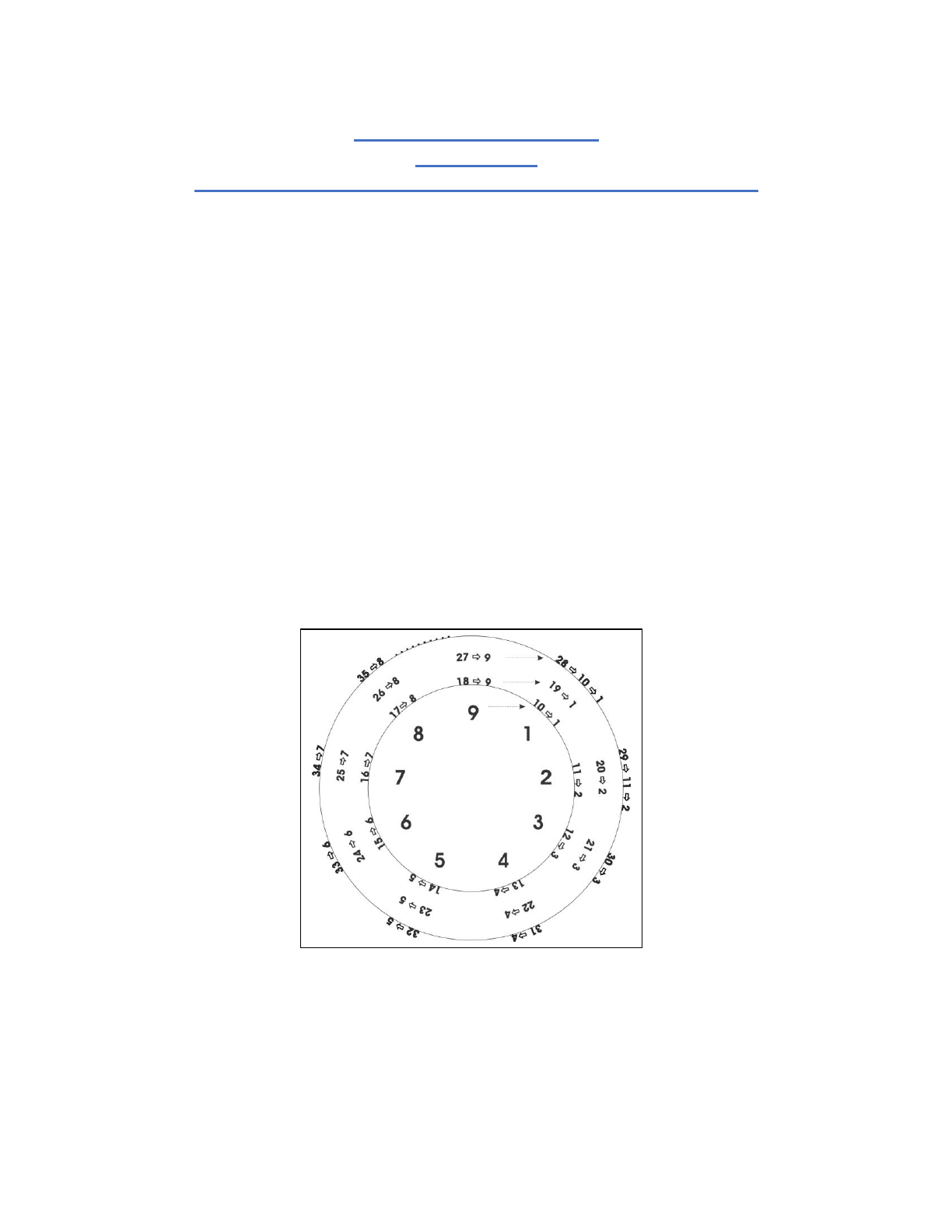Page 4 of 36 THE 3, 6 & 9 TRIUNE HIDDEN IN EVOLUTIONS ELEMENTARY BUILDING BLOCKS Since the Hindu-Arabic numbering system has played a unique role in the mathematics associated with evolution, we begin our search for the hidden 3, 6 & 9 Triune by examining the attributes of this system. First, it is the most efficient numbering system as it requires the fewest symbols to represent numbers. Second, by being based on ten digits (0-9), and thus a decimal system, its applicability is unusually flexible. While other numbering systems are based on increments of 10, the Hindu-Arabic system positions its digits in such a way that each multi-digit number can be added and re-added to form a repeating pattern of single-digit equivalents (i.e., 1-9), as shown below in Figure 1. Specifically, the multiple-digit number 10 can be summed to 1+0=1, 11 to 1+1=2, 12 to1+2=3, and so forth. As a result, when Hindu-Arabic numbers are arranged sequentiallyaround a set of concentric circles, the single-digit equivalents of each number form aspoke-like pattern, as shown in Figure 1. Figure 1. Consecutive Hindu-Arabic numbers reduce to concentric circles of nine single-digit sums or single-digit equivalents In other words, any multi-digit Hindu-Arabic number (that is not an endlessly repeating irrational decimal) can be expressed as one of the nine single-digit equivalents (i.e., 1-9) that forms the innermost circle in Figure 1, which is repeated in Figure 2 below. For consistency, all of this figures digits are evenly spaced geometrically because they are evenly spaced numerically (i.e., in increments of 1). HINDU-ARABIC NUMBERS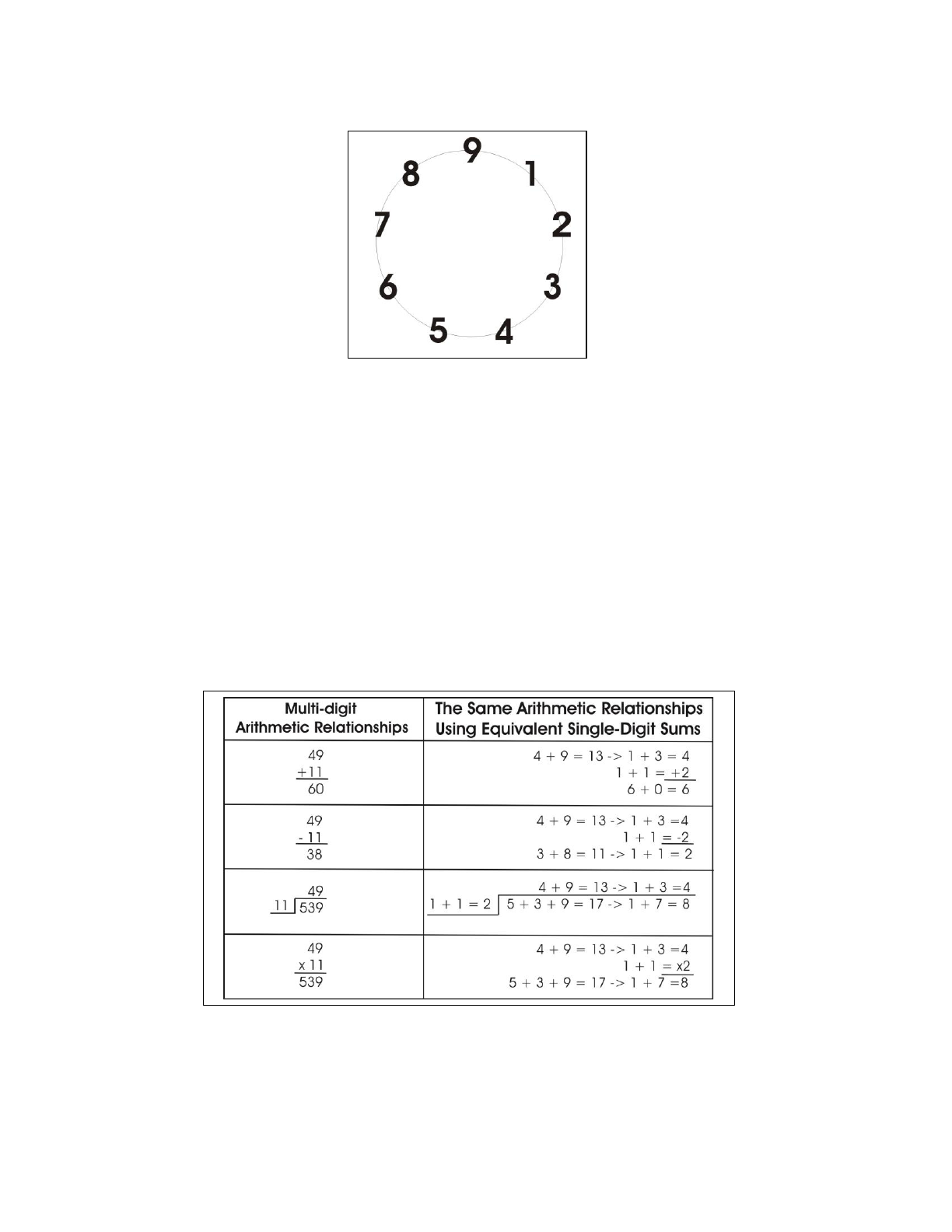Page 5 of 36 Figure 2. Circle of nine single-digit equivalents, 1-9 Also, by expressing all multi-digit numbers as single-digit equivalents (1 through 9), we can check basic arithmetic relationships between two Hindu-Arabic numbers involving addition, subtraction, division, and multiplication. To do so, we re-express arithmetic relationships between any two numbers as the same arithmetic relationships between their single-digit equivalents. Consider the numbers 11 and 34. The single-digit equivalents for these numbers are 2 and 7: 1+1=2 and 3+4=7. Note the arithmetic relationship of addition between these two numbers is 11+34=45. The single-digit equivalent of this sum is 4+5=9. This is exactly the answer from performing the same arithmetic operation  addition  on the single-digit equivalents of our original numbers: 2+7=9. Figure 3 provides several examples of this process. Figure 3. Using single-digit equivalents to check simple arithmetic While simple arithmetic relationships involving addition, subtraction, and multiplication between multi-digit numbers can be expressed in terms of the same arithmetic relationships between their single-digit equivalents (1-9), only a limited portion of the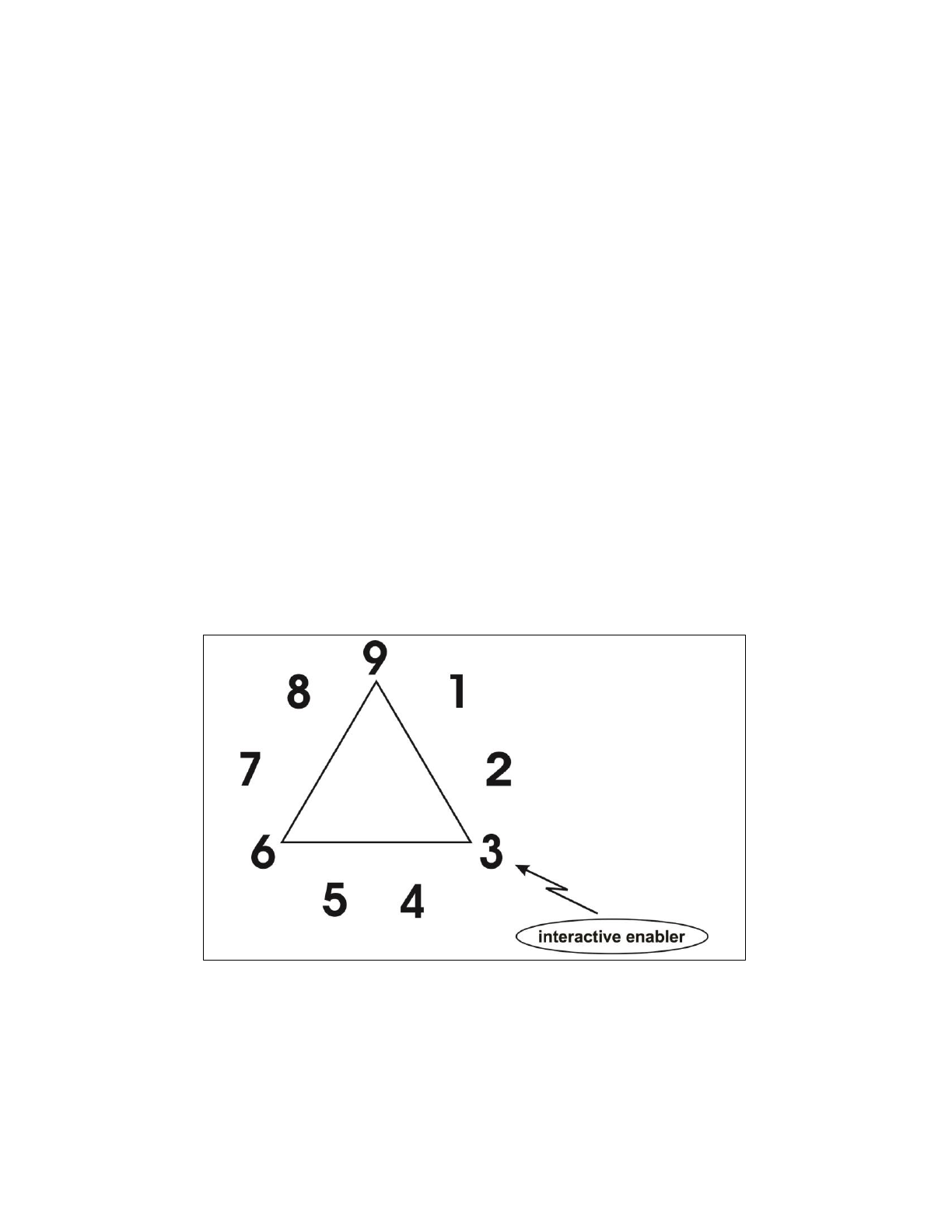Page 6 of 36 arithmetic relationships involving division can be similarly expressed. However, division can be checked by reversing the operation through multiplication. This overall process can be further simplified by casting out digits that sum to 9 before establishing the single-digit equivalents. For example, in Figure 3, the 9 in 49 could have been cast out and the single-digit equivalent of 49 would still be 4. Thus, this process has become known as casting out nines. In sum, the nine single-digit equivalents making up Figure 2 can effectively represent the entire Hindu-Arabic numbering system for basic arithmetic purposes. 3  interactive enabler The 3, 6 and 9 triangle in Figure 4 below is intended to illustrate that of the nine digits (i.e., 1-9) from Figure 2, 3 can only divide into 3, 6 and 9 and produce a single-digit equivalent answer without an endlessly repeating decimal. As a result, 3, 6 and 9 also cannot divide into any of the other six digits and produce a single-digit equivalent answer. Said another way, as the interactive common divisor of only 3, 6 and 9, 3 enables their differentiating indivisibility into the other six digits. Because of this unifying differentiation of the 3, 6 and 9 triangle, it will be referred to as the 3, 6 & 9 Triune, as shown in Figure 4. Figure 4. 3 as the interactive enabler of the 3, 6 & 9 Triune Also, three represents the number of digits in the 3, 6 & 9 Triune which, as we saw, was interactively enabled by 3. Further, note that the average value (expressed as a single-digit equivalent) of every pair of digits in the 3, 6 & 9 Triune is always equal to the other or third digit which also spatially bisects the respective pair on the circle of nine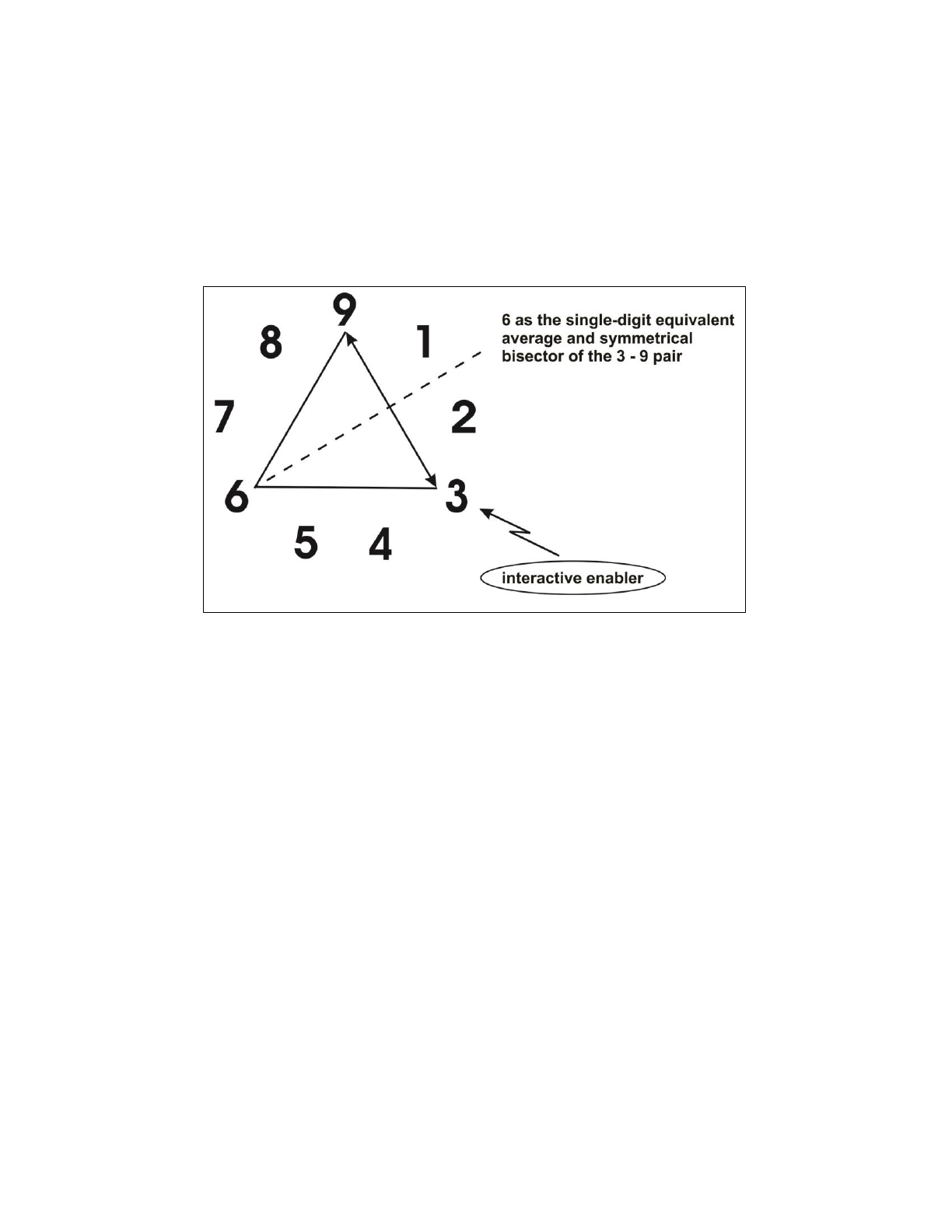Page 7 of 36 digits in Figure 4. For example, the 3 and 9 pair of digits have an average value of 6 [i.e., (3+9)/2 = 12/2 = 6], which is the third digit of the 3, 6 & 9 Triune and also spatially bisects the 3 and 9 pair on the circle of nine digits, as shown below in Figure 4a. As a result, 3 and 9 can be viewed as capable of emulating 6. Figure 4a. 6 is the single-digit equivalent average and symmetrical bisector of the 3-9 pair, thus making the latter capable of emulating the former Importantly, the 3, 6 & 9 Triune shares this axiom of spatial symmetry with every possible pair of digits in Figure 4 in that the average value of any pair (expressed as a single-digit equivalent) is always equal to the digit that spatially or symmetrically bisects the respective pair, thus making the latter capable of emulating the former. For example, the 8 and 5 pair of digits in Figure 5 below has an average single-digit equivalent value of 2 [i.e., (8+5)/2 = 13/2 = 6.5 => 6+5 = 11=> 1+1 = 2], which also spatially or symmetrically bisects the 8 and 5 pair on the circle of nine digits. To recap, the symmetrically bisecting process of the 3, 6 & 9 Triune that was interactively enabled by 3 is valid throughout the sequential nine-digit circle. Also, as noted, this circle of nine sequential single-digit equivalents can arithmetically substitute for all Hindu-Arabic numbers, as was illustrated in Figure 1.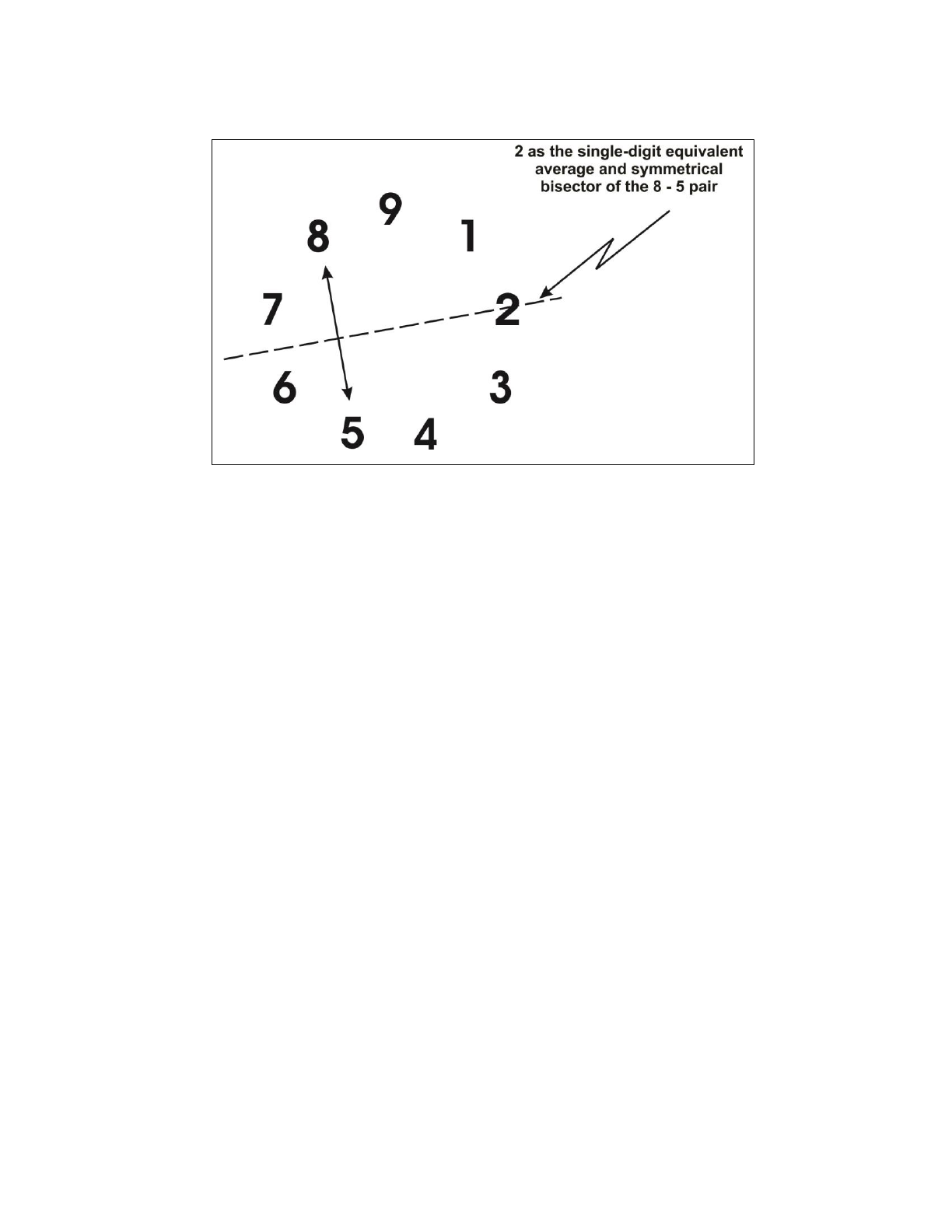Page 8 of 36 Figure 5. 2 is the single-digit equivalent average and the symmetrical bisector of the 8-5 pair, thus making the latter capable of emulating the former 6  integrative guidance Like 3, 6 is a member of the 3, 6 & 9 Triune, but six also represents the number of digits that are not part of the 3, 6 & 9 Triune (i.e., 1, 2, 4, 5, 7 and 8) in the circle of nine single-digit equivalents. Thus, it falls upon 6 to provide guidance for integrating the six digits outside the 3, 6 & 9 Triune (i.e., the non-triune digits) with the 3, 6 & 9 Triune digits. Since it was shown above that the symmetrical bisecting process of the 3, 6 & 9 Triune is valid throughout the sequential nine-digit circle, the six non-triune digits can be viewed as three different pairs symmetrically bisected by the 3, 6 & 9 Triune digits for a total of nine bracketing pairs, as shown below in Figure 6. Thus, the 3, 6 & 9 Triune digits serve as the single-digit equivalent averages and symmetrical bisectors of the bracketing 1, 2, 4, 5, 7 and 8 non-triune digits. As such, we can view the six non-triune digits as capable of emulating the 3, 6 & 9 Triune digits through the nine bracketing pairs, according to the integrative guidance represented by 6.